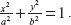## Asymptotes and Curve Tracing

The aim of this chapter is to study the shape of a plane curve y=f(x). For this purpose, we must investigate the variation of the function f, in the case of unlimited increase and absolute value and of x or y, or both, of a variable point (x, y) on the curve. The study of such variation of the function requires the concept of an asymptote. Before defining an asymptote to a curve, let us define finite and infinite branches of a plane curve as follows:

Consider the equation of the ellipseSolving this equation, we get

The first equation represents the upper half of the ellipse while the second equation represents ...

Get Engineering Mathematics, Volume I, Second Edition now with the O’Reilly learning platform.

O’Reilly members experience books, live events, courses curated by job role, and more from O’Reilly and nearly 200 top publishers.